Math Concepts

# How do you introduce Polynomials?

508 views

Table of Contents

 1 Introduction 2 What are polynomials? 3 Types of Polynomial 4 Degrees of Polynomials 5 Quadratic Polynomial 6 Factorization of Polynomial 7 Example Problems 8 Summary 9 About Cuemath 10 FAQs

28 January 2021

Reading time: 5 minutes

## Introduction

In mathematics, a polynomial is an expression that consists of variables and coefficients, involving the operations of addition, subtraction, multiplication, and exponentiation of variables.

For example: x2 − 4x + 7.

The word "Polynomial" contains two words, namely, “Poly" and “Nomials”. ‘Poly’ means many, and ‘nomials’ means terms. Hence an expression containing many terms is called Polynomials, having variables and coefficients. E.g. 3x, 5y2

### How do you introduce Polynomials-PDF

In this article, we will learn about polynomials, types of polynomials, degrees of polynomials, quadratic polynomials, factorization of polynomials. We will also see some examples. Here is a downloadable PDF to explore more.

 📥 How do you introduce Polynomials-PDF Download

Also read:

## What Are Polynomials?

Polynomials are expressions with one or more terms having a non-zero coefficient. These terms comprise variables, exponents, and constants.

A standard polynomial is one where the highest degree is the first term, and subsequent terms are arranged in ascending order based on the powers or the exponents.

Polynomials are classified based on the number of terms present in the expression as a monomial, binomial, and trinomial, which we will see in detail under types of polynomials.

Other terms related to polynomials are constants, variables, and exponents. Examples of each of them are shown below:

• Constants (E.g. 1, 2, 3, etc.)
• Variables (E.g. g, h, x, y, etc.)
• Exponents (E.g. 5 in x5)

### Applications

In real life, Polynomial functions are applied

• To design roller coaster rides.
• To build models of various buildings and objects, industries, construction, etc.
• In marketing, finance, stocks, etc.
• In different fields of science, such as physics, where we measure acceleration, or to express units of energy, inertia, or even electricity.
• In writing down the chemical equations.

## Types of Polynomials

There are different types of polynomials. Based on the number of terms, polynomials are classified as:

• Monomials
• Binomials
• Trinomials

A monomial is a polynomial expression that contains variables and a coefficient without addition or subtraction.

For example, 2x + 5x + 10x is a monomial because when we add the like terms it results in 17x. Furthermore, 4t, 21x2y, 9pq are also monomials.

A binomial is a polynomial with two unlike terms.

For example, 3x + 4x2 is binomial as it contains two unlike terms, that is, 3x and 4x2. Likewise, 10pq + 13p2q is also a binomial.

A trinomial is a polynomial with three unlike terms.

For example- 3x + 5x2 – 6x3 is a trinomial.

Likewise, 12pq + 4x2 – 10 is also a trinomial.

## Degrees of Polynomials

The highest power of the variable in a polynomial is called the degree of the polynomial. For example, in the following equation: x2+2x+4. The degree of the polynomial is 2 i.e. the highest power of the variable in the polynomial.

Example: Find the degree of 8x – 9

In this given example, the first term is 8x, whereas the second term is –9. Now, let us define the exponent for each term. The exponent of the first term 8x is 1 and of the second term –9, it is 0. Since the highest exponent is 1, the degree of 8x – 9 is also 1.

For a multivariable polynomial, it is the highest sum of powers of different variables in any of the terms in the expression.

For example: x5+3x4y+2xy3+4y2-2y+1.

It is a multivariable polynomial in x and y, and the degree of the polynomial is 5, as we can see the degree in the terms x5 is 5 , which is the highest degree among these individual terms .

Polynomials are also classified based on their degree. The different types are:

• Linear polynomial – of degree one
• Zero polynomial(constant) – of degree zero
• Quadratic Polynomial – of degree two
• Cubic Polynomial – of degree three

Exponents of variables of a polynomial i.e. degree of polynomials should be whole numbers.

There are 4 simple steps to find the degree of a polynomial which is explained in the steps listed below.

Example:

6x5+8x3+3x5+3x2+4+2x+4

• Step 1: Combine all the like terms that are the terms of the variable terms. (6x5+3x5)+8x3+3x2+2x+(4+4)
• Step 2: Ignore all the coefficient x5+x3+x2+x+x0
• Step 3: Arrange the variable in descending order of their power x5+x3+x2+x+x0
• Step 4: The largest power of the variable is the degree of the polynomial deg(x5+x3+x2+x+x0) = 5

## Quadratic Polynomial

A quadratic polynomial is a polynomial of degree 2.

• The zeroes of a polynomial p(x) are the x-coordinates of the points, where the graph of y = p(x) intersects the x-axis.

• A quadratic polynomial can have at the most 2 zeros.

• If α and β are the zeros of the quadratic polynomial ax2 + bx + c, then sum of the zeros:

$α +β = − \frac{b}{a}$

• If α and β are the zeros of the quadratic polynomial ax2 + bx + c, then product of the zeros: αβ = c/a

• The division algorithm states that given any polynomial p(x) and any non-zero polynomial g(x), there are polynomials q(x) and r(x) such that p(x) = g(x) q(x) + r(x), where r(x) = 0 or deg r(x) < deg g(x).

Example:

Factorize x2+8x+12

Solution:

Below the steps are given for your understanding

• Step 1: Factorize 12 as 12 = 2 × 6 or = 4 × 3. We have to find a pair, such that their product is equal to 12 and the sum is equal to 8. Only one such pair is possible,

i.e. 2 and 6. 2+6=8; 2*6=12.

• Step 2: Break middle term in terms of the summation of a pair of numbers such that its product is equal to 12 in the above case. We will write 8=6+2

x2+ (6+2)x+ 12 = x2+ 6x +2x + 12

• Step 3: Form pairs of terms and factor out GCF of the two pairs separately.

= x2+ 6x +2x + 12 = (x2+ 6x) +(2x + 12) = x(x+6)+2(x+6)

• Step 4: Again factor out GCF of the remaining sum of products. Follow the factorization procedure of binomials as explained earlier. Factor out (x+6) from sum of product =x(x+6)+2(x+6) = (x+6)(x+2)

## Factorization of Polynomials

The process involved in breaking a polynomial into the product of its factors is known as the factorization of polynomials.

It is similar to writing a factor tree and selecting the best combination to find the factors of the polynomial.

So when you factorize, find out a possible set of factors. E.g. One set of factors, of 24 is 6 and 4 because of 6 times 4 = 24. When you have a polynomial, one way of solving it is to factorize it into the product of two binomials.

The following are the steps to factorize a polynomial.

1. Check for the GCF first. ...

2. Multiply the quadratic term and the constant term. ...

3. Write down all the factors of the result, in pairs. ...

4. From this list, find the pair that adds to produce the coefficient of the linear term.

Let’s take an example: x2+10x+24

Since the coefficient of x2 is 1, we can assume that in both the linear factors, x will have a coefficient of 1. Thus, we have to find integers a and b such that

x2+10x+24 = (x+a)(x+b) = x2+(a+b)x+(a*b)

Comparing the coefficients on both sides, we have

a+b=10 ; ab=24

We thus have to figure out two integers whose sum is 10 and the product is 24. A little thinking shows that we can take

a=6 ; b=4

Accordingly, we split the middle term and obtain the two factors:

x2+10x+24 = x2+6x+ 4x+24

x(x+6)+4(x+6)

(x+4)(x+6)

So this way we can factorize the polynomial.

Algebraic Identities

• (x + y)2 = x2 + 2xy + y2
• (x – y)2 = x2 – 2xy + y2
• x2 – y2 = (x + y)(x – y)
• (x + a)(x + b)= x2 + (a + b)x + ab
• (x + y + z)2 = x2 + y2 + z2 + 2xy + 2yz + 2zx
• (x + y)3 = x3 + y3 + 3xy(x + y)
• (x – y)3 = x3 – y3 – 3xy(x – y)
• x3 + y3 + z3 – 3xyz = (x + y + z)(x2 + y2 + z2 – xy – yz – zx)
• If x + y + z = 0, then x3 + y3 + z3 = 3xyz

## Examples of Basic Problems

Here are some examples with answers to try your hands on:

Question 1:

The value of k for which (x+2) is a factor of (x+1)7+(3x+k)3 is :

 (A) −7 (B) 7 (C) -1 (D) −6−3(7/3)

Ans: B

Explanation:

Let p(x) = (x+1)7 +(3x+k)³

If (x+2) is a factor of p(x) then

p(-2) = 0

/* Factor theorem */

\begin{align}(-2+1) 7+[3(-2)+k]^{3}&=0 \-1)^{7}+(-6+{k})^{3}&=0 \\-1+(k-{6})^{3}&=0 \\(k-{6})^{3}&=1^{3} \\k-6&=1 \\k&=1+6 \\k&=7\end{align} Therefore, k = 7 Question 2 The value of (x−a)3+(x−b)3+(x−c)3−3(x−a)(x−b)(x−c), when a+b+c=3x is:  (A) 3 (B) 2 (C) 1 (D) 0 Ans: D Explanation: Take the identity: x3 + y3 + z3 – 3xyz = (x + y + z)(x2 + y2 + z2 – xy – yz – zx) and use it in the above problem. Consider ‘x’ as (x-a), ‘y’ as (x-b) and ‘z’ as (x-c), and substitute it in the above identity. We get : (x−a)3+(x−b)3+(x−c)3−3(x−a)(x−b)(x−c) = ({x-a} + {x-b} + {x-c})({x−a}2+{x−b}2+{x−c}2 - {x−a}{x−b} - {x−b}{x−c} - {x−c}{x−a}) On simplifying, (x−a)3+(x−b)3+(x−c)3−3(x−a)(x−b)(x−c) = (3x - {a+b+c})({x−a}2+{x−b}2+{x−c}2 - {x−a}{x−b} - {x−b}{x−c} - {x−c}{x−a}) We know that a+b+c = 3x. Substituting this in the above equation, (x−a)3+(x−b)3+(x−c)3−3(x−a)(x−b)(x−c) = (3x - {3x})({x−a}2+{x−b}2+{x−c}2 - {x−a}{x−b} - {x−b}{x−c} - {x−c}{x−a}) => (x−a)3+(x−b)3+(x−c)3−3(x−a)(x−b)(x−c) = (0)({x−a}2+{x−b}2+{x−c}2 - {x−a}{x−b} - {x−b}{x−c} - {x−c}{x−a}) = 0 Therefore, (x−a)3+(x−b)3+(x−c)3−3(x−a)(x−b)(x−c) = 0 Question 3 The remainder when x4−y4 is divided by x−y is ____.  (A) 0 (B) x+y (C) x2+y2 (D) 2y4 Ans: A Explanation: (x4−y4) can be simplified in the following way: (x4−y4) = (x2-y2)(x2+y2) (x4−y4) = (x+y)(x-y)(x2+y2) When divided by (x-y), {(x4−y4) / (x-y)} = { (x+y)(x-y)(x2+y2) / (x-y)} = (x+y)(x2+y2) Here, (x+y)(x2+y2) is the quotient, which when multiplied with the divisor (x-y), we get the dividend (x4−y4). Hence, the remainder here is 0. Question 4 If (x+2) and (x−1) are factors of (x3+10x2+mx+n) , then the value of m, n respectively are ____.  (A) −5,5 (B) 7,18 (C) 7,−18 (D) −5,−18 Ans: C Explanation: (x+2) is a factor of p(x)=(x3+10x2+mx+n) Therefore, by factor theorem, p(-2)=0 Therefore, p(-2)=({-2}3+10{-2}2+m{-2}+n)=0 On simplifying, we get : 2m-n=32 ……….(1) (x-1) is a factor of p(x)=(x3+10x2+mx+n) Therefore, by factor theorem, p(1)=0 Therefore, p(1)=({1}3+10{1}2+m{1}+n)=0 On simplifying, we get : m+n=(-11) ……….(2) Solving equation (1) and (2) we get m=7 and n=(-18) Question 5 What should be subtracted from f(x) = 6x3+11x2−39x−65 so that f(x) is exactly divisible by x2+x−1 ?  (A) 38x +60 (B) −38x−60 (C) −19x−30 (D) 9x+10 Ans: B Explanation: By long division method, we have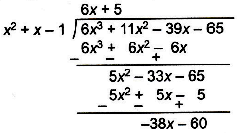We must subtract the remainder so that f(x) is exactly divisible by (x2 + x - 1). Hence, we should subtract (-38x - 60) from f(x). Question 6 The area of a triangular field is (x4−6x3−26x2+138x−35)m2 and the base of the triangular field is (x2−4x+1)m. Find the height of the triangular field.  (A) 2(x2−2x−35)m (B) 1/2(x2−2x−35)m (C) 2(3x2−x−4)m (D) 1/2(3x2−x−4)m Ans: A Question 7 Vikas has ₹(x3+2ax+b), with this money he can buy exactly (x−1) jeans or (x+1) shirts with no money left. How much money does Vikas have, if x=4?  (A) ₹80 (B) ₹120 (C) ₹30 (D) ₹60 Ans: D ### Advanced Level Problems Question 1 Obtain all the zeroes of the polynomial f(x)=3x4+6x3−2x2−10x−5 if two of its zeros are \(\frac{\sqrt5}{3} and $$-\frac{\sqrt5}{3}$$

 (A) 1 , −1 (B) 1 , 1 (C) −1 , −1 (D) 1 , 0

Ans: C

Question 2

Which of the following graphs has more than three distinct real roots?

 (A)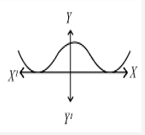(B)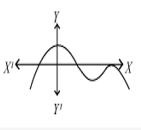(C)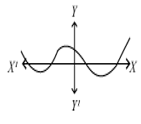(D)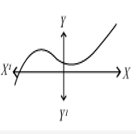Ans: C

Question 3

If $$x=\frac{a-b}{a+b}, \;y=\frac{b-c}{b+c}, z=\frac{c-a}{c+a}$$ find the value of $$\frac{(1+x)(1+y)(1+z)}{(1-x)(1-y)(1-z)}$$ is_________.

 (A) abc (B) a2b2c2 (C) 1 (D) -1

Ans: C

## Summary

Following are the main points covering what is polynomials are:

• Polynomials are expressions with one or more terms with a non-zero coefficient. A polynomial can have more than one term. These terms comprise variables, exponents, and constants.
• We can add and subtract polynomials by combining like terms.
• To multiply polynomials, use the distributive property to multiply each term in the first polynomial by each term in the second. Then add the products.

### Important terms used in Polynomials:

1. Polynomial- a sum of terms each consisting of a variable raised to a non-negative integer power

2. Monomial - a polynomial having one term

3. Binomial - a polynomial having two terms

4. Trinomial -a polynomial having three terms

5. Coefficient - any real number in a polynomial of the form anxn+⋯+a2x2+a1x+a0

6. Degree - the highest power of the variable that occurs in a polynomial

7. Leading term - the term containing the highest degree

8. Leading coefficient - the coefficient of the leading term

So, here we come to the end of the article with all the relevant points that are important for IMO. Don't forget to like and comment.

## About Cuemath

Cuemath, a student-friendly mathematics and coding platform, conducts regular Online Live Classes for academics and skill-development, and their Mental Math App, on both iOS and Android, is a one-stop solution for kids to develop multiple skills. Understand the Cuemath fee structure and sign up for a free trial.

## What is a polynomial equation?

The equations formed with variables, exponents, and coefficients are called polynomial equations.

## What is the degree of zero polynomial?

It is a constant polynomial whose all coefficients equals 0. The equivalent polynomial function is the constant function that has value 0, which we also call the zero map. Thus, the zero polynomial is said to be the additive identity of the additive group of polynomials.

## How to factorize a polynomial?

1. Check for the GCF first.
2. Multiply the quadratic term and the constant term.
3. Write down all the factors of the result, in pairs.
4. From this list, find the pair that adds to produce the coefficient of the linear term.

## What is a polynomial of five terms called?

Expressions having more than three terms are labeled by their number of terms. For instance, a five-term polynomial is a polynomial that has five terms.

## Who invented polynomials?

Rene Descartes invented polynomials. He is one of those who were responsible for introducing the concept of the graph of polynomial equations in 1637 in La geometric. Moreover, before this, there was a multiplication of polynomials happening in the 15th century where they wrote equations in words. For example, an algebra problem in circa 200 BCE, begins " Three sheaves of good crop, two sheaves of mediocre crop, and one sheaves of bad crops are sold for 29 dollar." We would write 3x + 2y + z = 29.

He also introduced different letters for constants, variables and exponents, for example, ax2 + bx = c is the general formula for a polynomial, where the a's denote constants and x denotes a variable. Descartes introduced the use of superscripts to denote exponentsas well.

Written by Monal Mashru, Cuemath Teacher

Related Articles
GIVE YOUR CHILD THE CUEMATH EDGE
Access Personalised Math learning through interactive worksheets, gamified concepts and grade-wise courses
Learn More About Cuemath